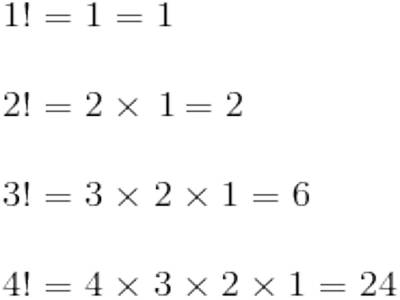Factorials

In mathematics, the factorial of a non-negative integer n, denoted by n! is the product of all positive integers less than or equal to n. A factorial is designated by a positive whole number followed by an exclamation point (e.g. 8!). The value of a factorial is the product of all the numbers from 1 through the number of the factorial. 8! = 1*2*3*4*5*6*7*8 = 40,320. Factorials are used in determining the numbers of combinations and permutations and in finding the probability.

The factorial operation is encountered in many areas of mathematics, notably in combinatorics, algebra, and mathematical analysis. Its most basic occurrence is the fact that there are n! ways to arrange n distinct objects into a sequence (i.e., permutations of the set of objects). This fact was known at least as early as the 12th century, to Indian scholars. Fabian Stedman, in 1677, described factorials as applied to change ringing.

Factorials are very simple things. They’re just products, indicated by an exclamation mark. For instance, “four factorial” is written as “4!” and means 1×2×3×4 = 24. In general, n! (“end factorial”) means the product of all the whole numbers from 1 to n; that is, n! = 1×2×3×…×n.Factorial Problem 1

How many different ways can the letters in the word “document” be arranged?

For this problem, we simply take the number of letters in the word and find the factorial of that number. This works because each letter in the word is unique and we are simply finding the maximum amount of ways 8 items can be ordered.

8!=8*7*6*5*4*3*2*1= 40,320

Factorial Problem 2

How many different ways can the letters in the word “physics” be arranged?

This problem is slightly different because there are two “s” letters. To account for this we divide by the number of duplicate letters factorial. There are 7 letters in the word physics and two duplicate letters so we must find 7!/2!. If the word had multiple duplicates, as in “little,” the formula would be 6!/(2! * 2!).

7!/2!=(7*6*5*4*3*2*1)/(2*1)= 2,520

Information Source;